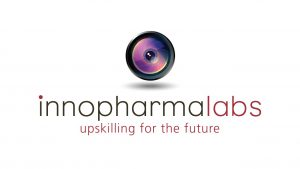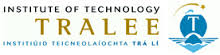``` ```
``````
``` jQuery( document ).ready(function(\$) { document.addEventListener("touchstart", function(){}, true); jQuery.fn.randomize = function (selector) { var \$elems = selector ? \$(this).find(selector) : \$(this).children(), \$parents = \$elems.parent(); \$parents.each(function () { \$(this).children(selector).sort(function (childA, childB) { // * Prevent last slide from being reordered if(\$(childB).index() !== \$(this).children(selector).length - 1) { return Math.round(Math.random()) - 0.5; } }.bind(this)).detach().appendTo(this); }); return this; }; var randomId = "117-651337939a40b"; var logoSlider = \$("#"+randomId); var slides = (logoSlider.innerHTML); //slides = slides.replace(/\s/g,""); slides = slides.replace(/(\r\n|\n|\r)/gm,""); var lazy_load = "0"; logoSlider.slick({ slidesToShow: 5, slidesToScroll: 1, infinite: false, pauseOnHover: false, variableWidth: false, autoplaySpeed: 4000, speed: 1000, rtl: false, arrows: false, asNavFor: null, autoplay: true, draggable: false, centerMode: false, centerPadding: '0px', useCSS: true, swipeToSlide: true, dots: false, }); }); ```